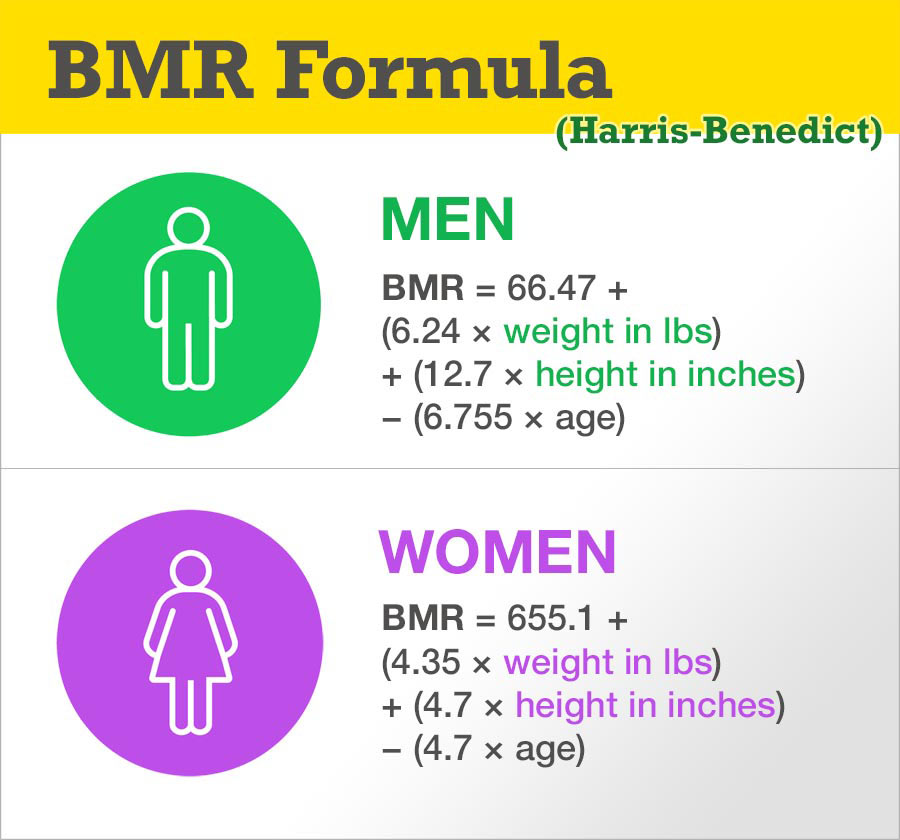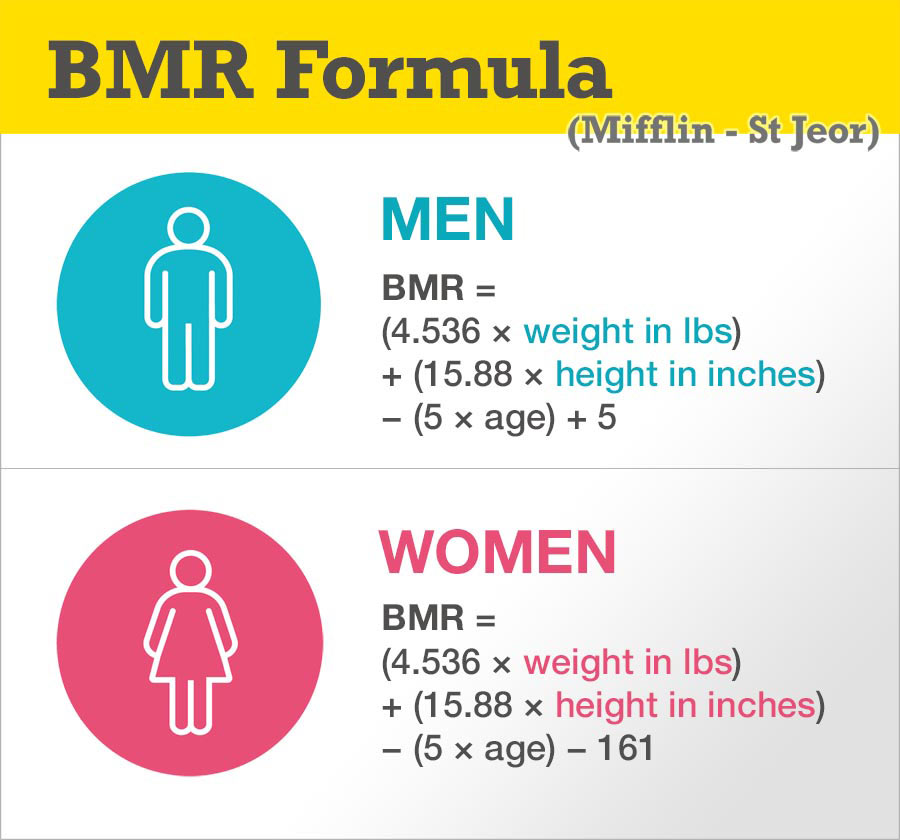# BMR Formula (Basal Metabolic Rate)

| Last update: 04 December 2018

BMR stands for Basal Metabolic Rate and represents your daily energy expenditure if your body is at rest all day. Look upon it as the minimum number of calories your body needs in order to function for 24 hours.

To calculate your BMR, you require an equation (commonly the Harris-Benedict formula or Mifflin-St Jeor formula). Into that, you enter your individual weight and height measurements and your age. Let's take a look at each BMR formula in turn.

## Harris-Benedict Equation

The original Harris-Benedict equation was created in 1919 following a study by James Arthur Harris and Francis Gano Benedict. The equations were revised in 1984 using new data, in order to improve accuracy. The Harris-Benedict equation was the primary, relied-upon formula for BMR calculations until 1990, when the Mifflin St Jeor equation was published.The Harris-Benedict BMR equation is as follows:

### Metric formula for men

BMR = 66.47 + ( 13.75 × weight in kg ) + ( 5.003 × height in cm ) − ( 6.755 × age in years )

### Imperial formula for men

BMR = 66.47 + ( 6.24 × weight in pounds ) + ( 12.7 × height in inches ) − ( 6.755 × age in years )

### Metric formula for women

BMR = 655.1 + ( 9.563 × weight in kg ) + ( 1.85 × height in cm ) − ( 4.676 × age in years )

### Imperial formula for women

BMR = 655.1 + ( 4.35 × weight in pounds ) + ( 4.7 × height in inches ) − ( 4.7 × age in years )

## Mifflin - St Jeor Equation

In 1990, a study by Mifflin MD and St Jeor was published in the American Journal of Clinical Nutrition. It discussed a new predictive equation for resting energy expenditure in healthy individuals, suggesting an update to the existing Harris-Benedict method. The Mifflin - St Jeor equation was said to be more predictive for modern lifestyles and has established itself as the standard for calculating BMR estimates. With that said, both equations are still in common use today.The Mifflin - St Jeor BMR equation is as follows:

### Metric formula for men

BMR = (10 × weight in kg) + (6.25 × height in cm) − (5 × age in years) + 5

### Imperial formula for men

BMR = (4.536 × weight in pounds) + (15.88 × height in inches) − (5 × age) + 5

### Metric formula for women

BMR = (10 × weight in kg) + (6.25 × height in cm) − (5 × age in years) − 161

### Imperial formula for women

BMR = (4.536 × weight in pounds) + (15.88 × height in inches) − (5 × age) − 161;

## Which BMR formula should I use?

Both the Harris-Benedict formula and Mifflin - St Jeor formula are commonly used and referenced today. It has been suggested that the difference between the Harris-Benedict and Mifflin-St Jeor equations is around 5%, with a higher accuracy level achieved by the Mifflin - St Jeor formula.

## Calculating your daily calorie requirement

After you've calculated your BMR, you may wish to work out your daily calorie requirement. Note that the BMR calculator can help calculate your BMR and daily calorie requirement for you (or check your manual calculation from above). Should you wish to calculate your daily calorie needs manually, simply multiply your BMR by one of the following activity level factors:

1. If you are sedentary (little or no exercise)
Calories Per Day = BMR x 1.2
2. If you are lightly active (light exercise or sports 1-3 days/week)
Calories Per Day = BMR x 1.375
3. If you are moderately active (moderate exercise 3-5 days/week)
Calories Per Day = BMR x 1.55
4. If you are very active (hard exercise 6-7 days/week)
Calories Per Day = BMR x 1.725
5. If you are super active (very hard exercise and a physical job)
Calories Per Day = BMR x 1.9

I hope this article has been of use to you. If you would like more explanation on the ins and outs of BMR, please visit our BMR calculator page.